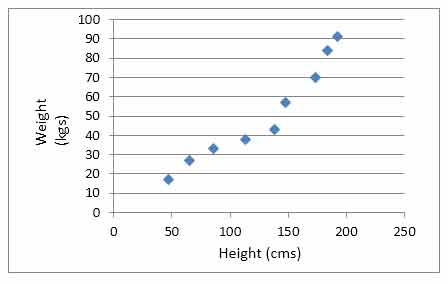# Scatter Plot

Posted in Statistics, Total Reads: 3292

## Definition: Scatter Plot

scatter plot, also known as scatter chart, scatter diagram, or scattergraph, is a type of mathematical chart which displays a set of data, as a collection of individual points, using two variables on the two Cartesian coordinates. Each point represents one data, showing the value of both the variables for that particular data. Scatter plot is one of the seven basic tools used in quality control. It is also used in finding out the future trends by studying past data.

The use of scatter plots is when there is a huge data set and the requirement is to obtain the relationship between two variables, if any exists. Unlike line graphs, here we get a scattered set of points which are used to get ‘best fit line’ or ‘trendline’. Closer the points to this line, stronger the correlation between the two variables.

Three scenarios emerge:

• Positive correlation – the slope of the line is from left to right (rising); as the value of one variable increases, the value of other also increases
• Negative correlation – the slope of the line is from right to left (falling); as the value of one variable increases, the value of other decreases
• No correlation – All points are scattered and no best fit line could be drawn

Example:

Correlation between height and weight of an individual can be found out using this data set:

 Height (cms) Weight (Kgs) 47 17 65 27 86 33 113 38 138 43 148 57 173 70 184 84 192 91

The corresponding scatter plot gives a positive correlation.Hence, this concludes the definition of Scatter Plot along with its overview.

Browse the definition and meaning of more terms similar to Scatter Plot. The Management Dictionary covers over 7000 business concepts from 6 categories. This definition and concept has been researched & authored by our Business Concepts Team members.

Search & Explore : Management Dictionary

Similar Definitions from same Category: